sk|cz|

# Triangle

1.Characterize a triangle

Solution: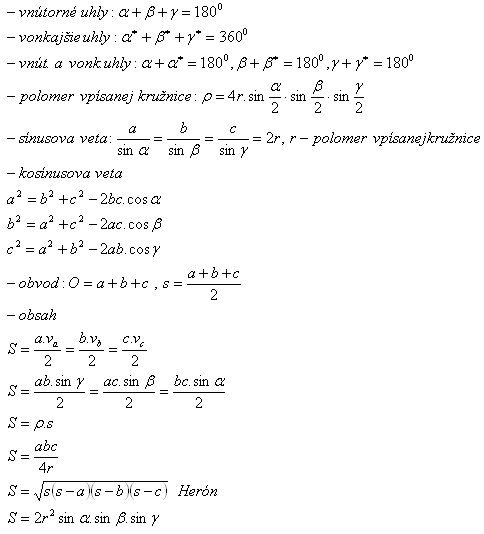2.Prove for the exterior angles of a triangle: α*+ β*+ γ* = 360°.

Proof:

α + α* = 180°
β + β* = 180°
γ + γ* = 180°
_____________

α + β + γ + α* + β* + γ* = 540°
α* + β* + γ* = 540° – (α + β + γ)
α* + β* + γ* = 540° - 180°
α* + β* + γ* = 360°

3. A triangles‘ side size c = 10 cm. For it‘s angles stands: α:β:γ = 3:5:10.

Determine:
a) perimeter
b) area
Solution:

Perimeter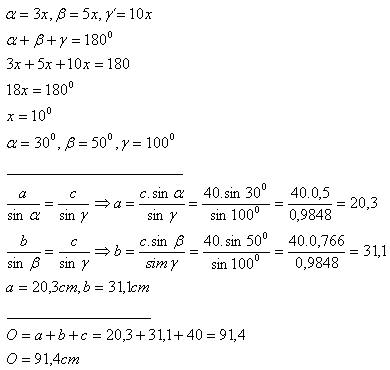Area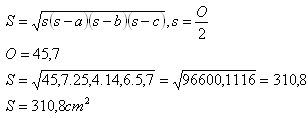4.An equilateral triangle‘s side length a = 10 cm.

Determine the size of the area between the circumcircle and the incircle.
Solution: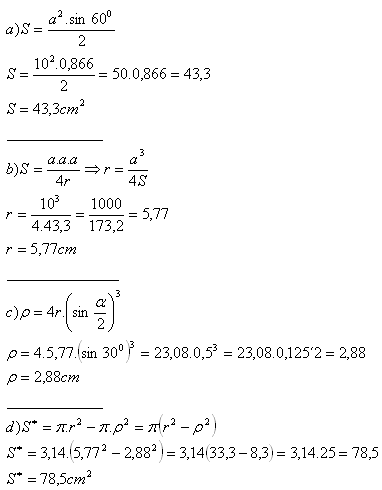5.John stands 5 m and 8 m from the edges of a tree. He can see the tree under the angle of 60°.

Determine the length of the tree.
Solution: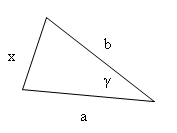a = 5 m
b = 8 m
γ = 60°

x2 = a2 + b2 -2ab.cosγ
x2 = 52 + 82 -2.5.8.cos60°
x2 = 25 + 64 – 80.0,5
x2 = 25 + 64 – 40
x2 = 49
x = 7

The tree is 7 m long.

6.There are three tangent circles with radius r1 = 2 cm, r2 = 3cm, r3 = 4cm. Centres of the circles define a triangle.

Determine the inner angles of the triangle.
Solution: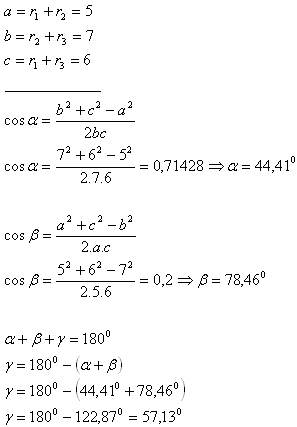Inner angles of the triangle equal α = 44,41°, β = 78,46° and γ = 57,13°.

7.Two trains left a train station in the same time on two tracs forming the angle φ = 156°. Their speeds are v1 = 13 ms-1, v2 = 14,5 ms-1.

Determine their distance in the time t = 5 min.
Solution: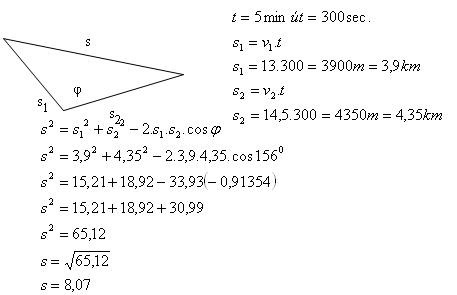The distance between the trains after 5 minutes is 8.07 km.

8. Forces F1, F2 act from the same point of application. Angle between the forces φ = 60°.

Determine the size of the resultant power, if F1 = 42N, F2 = 35N.
Determine the angles between the forces F1, F2 and the resultant power.

Solution: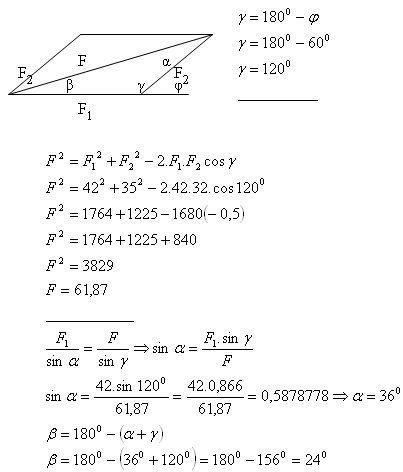The resultant power F = 61,87N.

The angles are α = 36° and β = 24°.

9.The angle between the coasts of a river observed from a 20 m height tower which is 20 m far from the river is 15°.

Determine the width of the river.
Solution: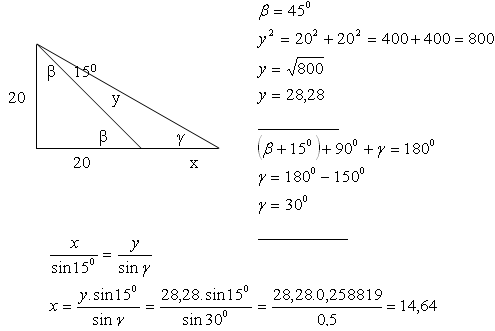The width of the river is x = 14,64 m.

10. An object of weight m = 1563 kg hangs on a horizontal girder. It‘s fastened by two ropes of different length. The angles between a ropes and the girder equal α = 48°, β = 62°.

Determine the forces acting on the ropes. (F = 15630 N)
Solution: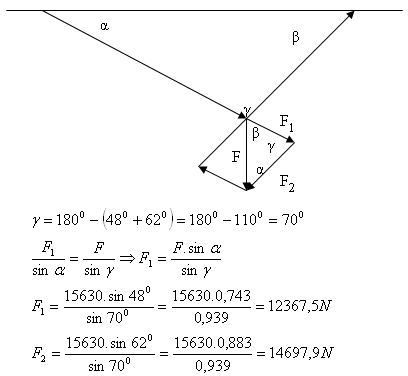The forces acting on the ropes are F1 = 12367,5 N and F2 = 14697,9 N.